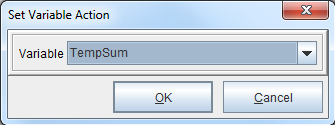## Latest News

the latest news from our team

# Math Calculations with Arrays

NOTE: This example makes use of the Calculation, Set Variable and Sum procedures. More information can be found in the following posts:
Calculation and Sum Procedures
Set Variable

To perform math calculations with an array, it’s necessary to create a variable that contains the array and then use the Calculation procedure to perform the required math functions. In the examples listed below, the Calculation procedure is used to multiply a set of values. In one example, the Sum procedure is used to add the total to a separate variable. In another example, the Set Variable procedure is used. To follow along, download the files here: Calculation

## Method One: Sum Procedure

This example uses the project ‘Sum1’.

1. Add a variable containing the array of values.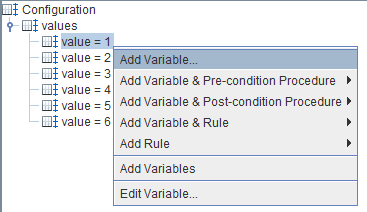2. Name the variable or accept the defaults. Select OK.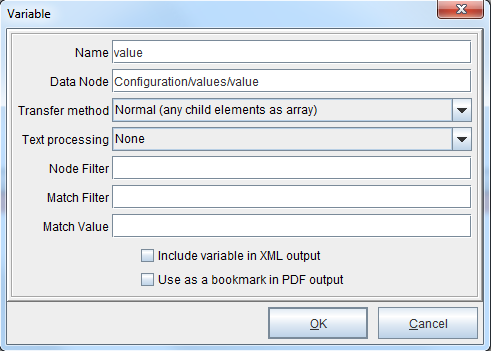3. Add a variable by position by right clicking the form and selecting Add Variable > By position. This variable will be used as the multiplier.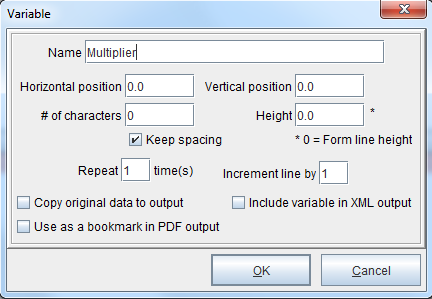4. Right click the multiplier variable and select Add Function > Set Value. In this instance, we will be using a multiplier of 3.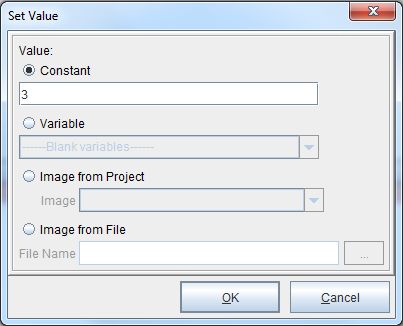5. Create a copy of the variable containing the array of values but give it a different name (‘TempValue’ in this example).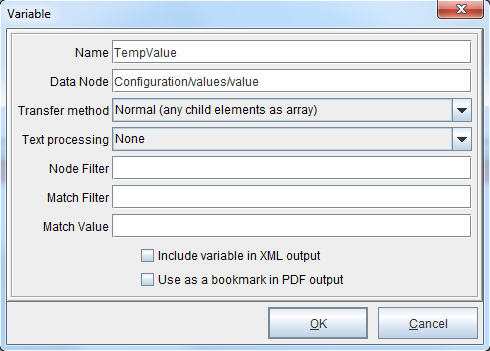6. Add a variable by position by right clicking the form and selecting Add Variable > By position. This variable will be used as the sum.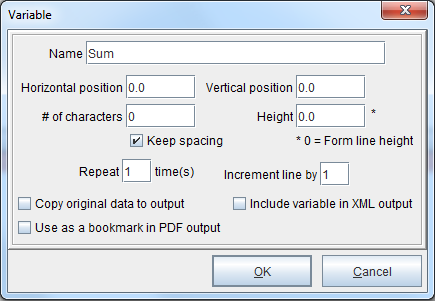7. Right click the form and select Add Pre-condition Procedure > Calculation. The Procedure Properties dialog box will display. Name the procedure (‘Multiply’ in this example). From the drop-down menu, select the variable which contains the array to calculate. Select OK.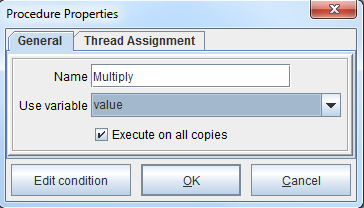8. The Calculate dialog box will display. Select Multiply from the drop-down menu. Next, select the Variable radio button and the variable which contains the multiplier. Select OK.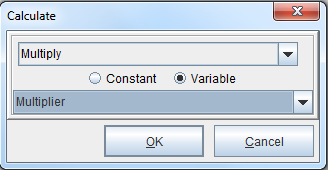9. Right click the procedure (which is named ‘Multiply’ in this example) and select Add Action > Set Variable. The Set Variable Action dialog box will display. From the Variable drop-down menu, select the ‘TempValue’ variable created earlier (See step #5).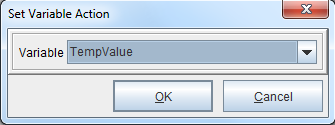10. Right click the form and select Add Pre-condition Procedure > Sum. The Procedure Properties dialog box will display. Select the ‘TempValue’ variable created earlier (See step #5). Select OK.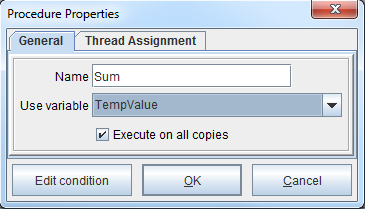11. The Sum into Variable Action dialog box will display. From the Variable drop-down menu, select the ‘Sum’ variable created earlier (See step #6). Select OK. The ‘Sum’ variable will now have the total.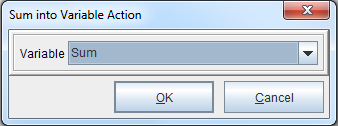## Method Two: Set Variable Procedure

This example uses the project ‘Sum2’.

1. Add a variable containing the array of values.2. Name the variable or accept the defaults. Select OK.3. Add a variable by position by right clicking the form and selecting Add Variable > By position. This variable will be used as the multiplier.4. Right click the multiplier variable and select Add Function > Set Value. In this instance, we will be using a multiplier of 3.5. Add a variable by position by right clicking the form and selecting Add Variable > By position. This variable will be used as the sum (‘TempSum’ in this example).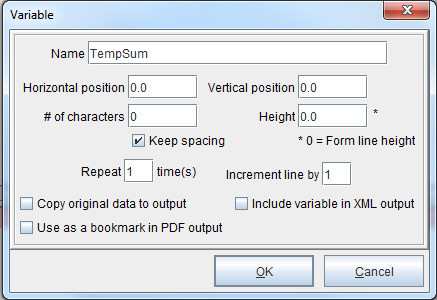6. Right click the form and select Add Pre-condition Procedure > Set Variable. The Procedure Properties dialog box will display. This procedure will preset the value of ‘TempSum’ to null. Select the N/A system variable from the drop-down menu.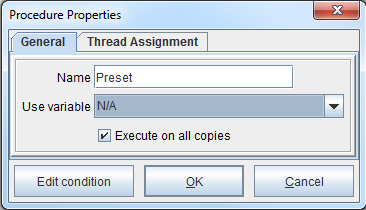7. The Set Variable Action dialog box will display. Select the ‘TempSum’ variable and select OK.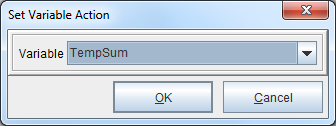8. Right click the Actions icon beneath the procedure and select Add Function > Set value.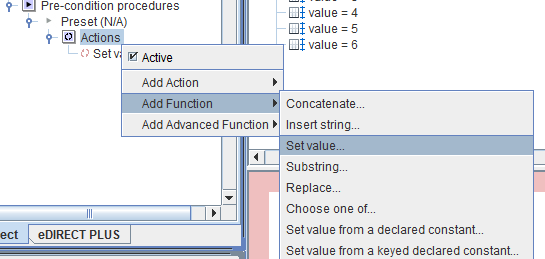9. The Set Value dialog box will display. Enter a value of ‘0’ in the Constant field and select OK.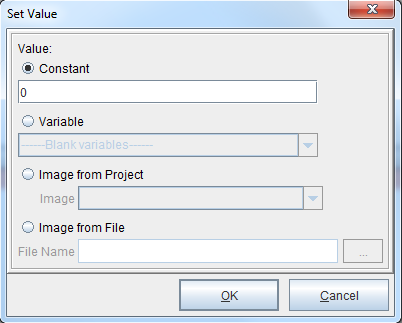10. In the Project window, left click the ‘Set value to 0’ function and drag it above the ‘Set variable TempSum’ procedure. This will set ‘TempSum’ to 0.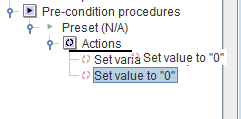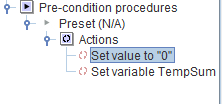11. Right click the form and select Add Pre-condition Procedure > Calculation. The Procedure Properties dialog box will display. Select the variable which contains the array to calculate (‘value’ in this example). Select OK.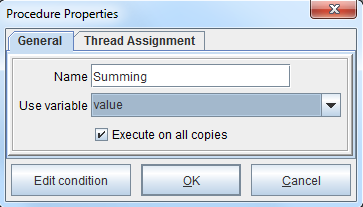12. The Calculate dialog box will display. Select the Multiply option and select the variable for the multiplier created earlier (See step #3). Select OK.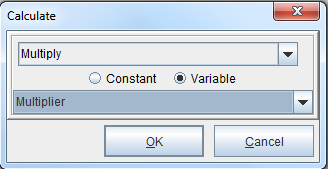13. Right click the Actions icon beneath the procedure and select Add Action > Calculation.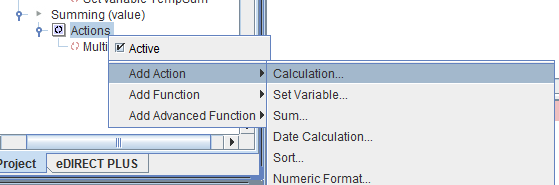14. Right click the Actions icon beneath the procedure and select Add Action > Calculation. Select the Add option and select the ‘TempSum’ variable. Select OK.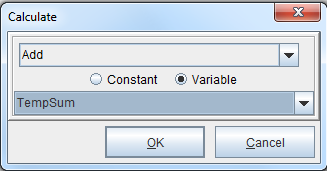15. Right click the Actions icon beneath the procedure and select Add Action > Set Variable.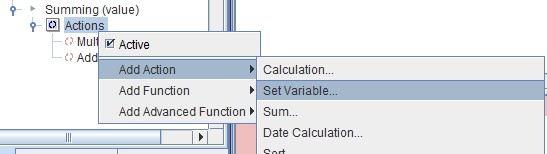16. The Set Variable Action dialog box will display. Select the ‘TempSum’ variable. Select OK. The ‘TempSum’ variable will now have the total.